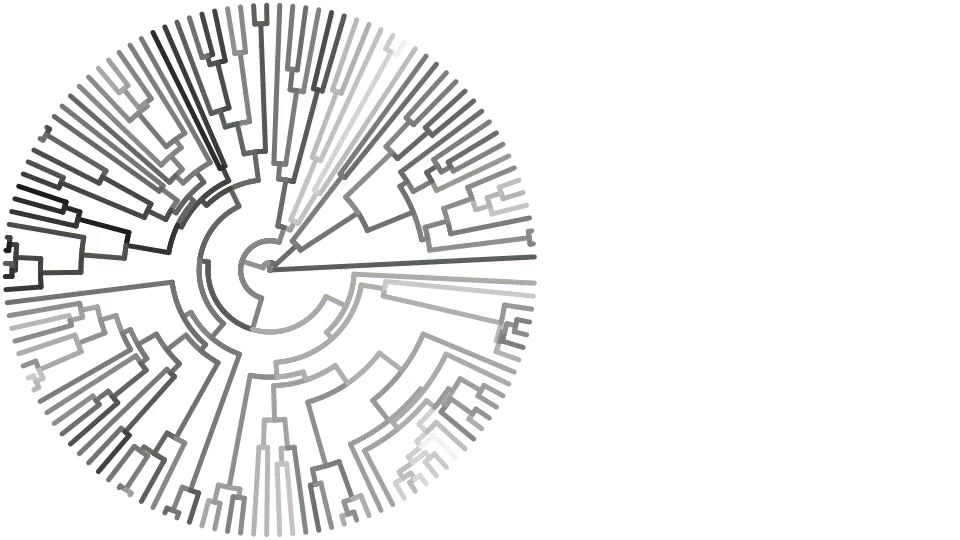using Makie, MakieRecipes

## The simplest example model

using MakieRecipes.RecipesBase

struct T end

RecipesBase.@recipe function plot(::T, n = 1; customcolor = :green)
markershape --> :auto        # if markershape is unset, make it :auto
markercolor :=  customcolor  # force markercolor to be customcolor
xrotation   --> 45           # if xrotation is unset, make it 45
zrotation   --> 90           # if zrotation is unset, make it 90
rand(10,n)                   # return the arguments (input data) for the next recipe
end

recipeplot(T(); seriestype = :path)

AbstractPlotting.save("basic.svg", AbstractPlotting.current_scene()); nothing #hide## Testing out series decomposition

sc = Scene()
recipeplot!(sc, rand(10, 2); seriestype = :scatter)
recipeplot!(sc, 1:10, rand(10, 1); seriestype = :path)

AbstractPlotting.save("series.svg", AbstractPlotting.current_scene()); nothing #hide## Differential Equations

using OrdinaryDiffEq, StochasticDiffEq, DiffEqNoiseProcess

### A simple exponential growth model

f(u,p,t) = 1.01.*u
u0 = [1/2, 1]
tspan = (0.0,1.0)
prob = ODEProblem(f,u0,tspan)
sol = solve(prob, Tsit5(), reltol=1e-8, abstol=1e-8)

recipeplot(sol)

AbstractPlotting.save("exp.svg", AbstractPlotting.current_scene()); nothing #hide### Matrix DiffEq

A  = [1. 0  0 -5
4 -2  4 -3
-4  0  0  1
5 -2  2  3]
u0 = rand(4,2)
tspan = (0.0,1.0)
f(u,p,t) = A*u
prob = ODEProblem(f,u0,tspan)
sol = solve(prob, Tsit5(), reltol=1e-8, abstol=1e-8)

recipeplot(sol)

AbstractPlotting.save("mat.svg", AbstractPlotting.current_scene()); nothing #hide### Stochastic DiffEq

f(du,u,p,t) = (du .= u)
g(du,u,p,t) = (du .= u)
u0 = rand(4,2)

W = WienerProcess(0.0,0.0,0.0)
prob = SDEProblem(f,g,u0,(0.0,1.0),noise=W)
sol = solve(prob,SRIW1())

recipeplot(sol)

AbstractPlotting.save("stochastic.svg", AbstractPlotting.current_scene()); nothing #hide### Animating a differential equation solution

You can update arguments and attributes arbitrarily, and the recipe pipeline is rerun.

function lorenz(du, u, p, t)
du = p*(u-u)
du = u*(p-u) - u
du = u*u - p*u
end

prob = ODEProblem(lorenz, [1., 5., 10.], (0., 100.), (10.0, 28.0, 8/3))

ρ = Node(28.0)

sol = @lift solve(remake(prob; p = (10.0, \$ρ, 8/3)), Tsit5())

sc = recipeplot(sol)

ylims!(sc, -30, 70) # avoid jitter when animating

record(sc, "lorenz.gif", LinRange(0, 35, 100)) do ρᵢ
ρ[] = ρᵢ
end## Phylogenetic tree

using Phylo

assetpath = joinpath(dirname(pathof(MakieRecipes)), "..", "docs", "src", "assets")
hummers = open(t -> parsenewick(t, NamedPolytomousTree), joinpath(assetpath, "hummingbirds.tree"))

evolve(tree) = Phylo.map_depthfirst((val, node) -> val + randn(), 0., tree, Float64)

trait = evolve(hummers)

scp = recipeplot(
hummers;
treetype = :fan,
line_z = trait,
linewidth = 5,
showtips = false,
cgrad = :RdYlBu,
seriestype = :path,
scale_plot = false, # Makie attributes can be used here as well!
show_axis = false
)
WARNING: using Phylo.Node in module ex-examples conflicts with an existing identifier.### Animation with different colormaps (changing attributes)

You can update arguments and attributes arbitrarily, and the recipe pipeline is rerun.

record(scp, "phylo_colormaps.gif", PlotUtils.cgradients(:colorcet), framerate = 3) do cmap
scp.plots.cgrad[] = cmap
end
"phylo_colormaps.gif"## GraphRecipes

using GraphRecipes
using GraphRecipes: GraphPlot

In tis case, we don't need access to all of the functions which GraphRecipes provides - just the GraphPlot struct, which is used for recipe dispatch. The rest is handled by the GraphRecipes internals.

### The simplest graph recipe

Because userplot is unsupported, we have to use the low-level interface to user plot recipes, by wrapping our arguments in the userplot type. Arguments must be supplied as a Tuple or some other iterable.

recipeplot(
GraphPlot(
(
[0  1  1;
1  0  1;
1  1  0],
)
);
show_axis = false,
scale_plot = false
)

AbstractPlotting.save("simplegraph.svg", AbstractPlotting.current_scene()); nothing #hide### Undirected graph with node labels and sizes

n = 15
A = Float64[ rand() < 0.5 ? 0 : rand() for i=1:n, j=1:n]
for i=1:n
A[i, 1:i-1] = A[1:i-1, i]
A[i, i] = 0
end

recipeplot(
GraphPlot((A,));
markersize = 0.2,
node_weights = 1:n,
markercolor = range(colorant"yellow", stop=colorant"red", length=n),
names = 1:n,
fontsize = 10,
linecolor = :darkgrey,
show_axis = false,
scale_plot = false
)

AbstractPlotting.save("undigraph.svg", AbstractPlotting.current_scene()); nothing #hide### Multigraphs

recipeplot(GraphPlot(([[1,1,2,2],[1,1,1],],)); names="node_".*string.(1:3), nodeshape=:circle, self_edge_size=0.25, show_axis = false, scale_plot = false)

AbstractPlotting.save("digraph.svg", AbstractPlotting.current_scene()); nothing #hide### Julia AST

code = quote
function mysum(list)
out = 0
for value in list
out += value
end
out
end
end

recipeplot(code; fontsize = 12, shorten = 0.01, axis_buffer = 0.15, nodeshape = :rect)
AbstractPlotting.save("ast.svg", AbstractPlotting.current_scene()); nothing #hide### Type tree with GraphRecipes

recipeplot(AbstractFloat; method = :tree, fontsize = 10)
AbstractPlotting.save("typetree.svg", AbstractPlotting.current_scene()); nothing #hideThis page was generated using Literate.jl.